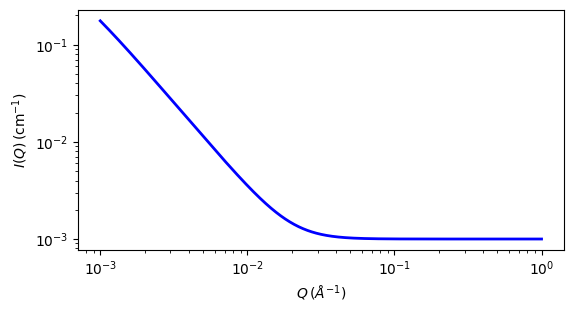# mass_surface_fractal

Mass Surface Fractal model

Parameter

Description

Units

Default value

scale

Scale factor or Volume fraction

None

1

background

Source background

cm-1

0.001

fractal_dim_mass

Mass fractal dimension

None

1.8

fractal_dim_surf

Surface fractal dimension

None

2.3

rg_cluster

4000

rg_primary

86.7

The returned value is scaled to units of cm-1 sr-1, absolute scale.

A number of natural and commercial processes form high-surface area materials as a result of the vapour-phase aggregation of primary particles. Examples of such materials include soots, aerosols, and fume or pyrogenic silicas. These are all characterised by cluster mass distributions (sometimes also cluster size distributions) and internal surfaces that are fractal in nature. The scattering from such materials displays two distinct breaks in log-log representation, corresponding to the radius-of-gyration of the primary particles, $$rg$$, and the radius-of-gyration of the clusters (aggregates), $$Rg$$. Between these boundaries the scattering follows a power law related to the mass fractal dimension, $$Dm$$, whilst above the high-Q boundary the scattering follows a power law related to the surface fractal dimension of the primary particles, $$Ds$$.

Definition

The scattered intensity I(q) is calculated using a modified Ornstein-Zernicke equation

$\begin{split}I(q) &= \text{scale} \times P(q) + \text{background} \\ P(q) &= \left\{ \left[ 1+(q^2a)\right]^{D_m/2} \times \left[ 1+(q^2b)\right]^{(6-D_s-D_m)/2} \right\}^{-1} \\ a &= R_{g}^2/(3D_m/2) \\ b &= r_{g}^2/[-3(D_s+D_m-6)/2] \\ \text{scale} &= \text{scale factor} \times NV^2 (\rho_\text{particle} - \rho_\text{solvent})^2\end{split}$

where $$R_g$$ is the size of the cluster, $$r_g$$ is the size of the primary particle, $$D_s$$ is the surface fractal dimension, $$D_m$$ is the mass fractal dimension, $$\rho_\text{solvent}$$ is the scattering length density of the solvent, and $$\rho_\text{particle}$$ is the scattering length density of particles.

Note

The surface ( $$D_s$$ ) and mass ( $$D_m$$ ) fractal dimensions are only valid if $$0 < surface\_dim < 6$$, $$0 < mass\_dim < 6$$, and $$(surface\_dim + mass\_dim ) < 6$$. Older versions of sasview may have the default primary particle radius larger than the cluster radius, this was an error, also present in the Schmidt review paper below. The primary particle should be the smaller as described in the original Hurd, et al., who also point out that polydispersity in the primary particle sizes may affect their apparent surface fractal dimension.Fig. 111 1D plot corresponding to the default parameters of the model.

Source

References

1. P Schmidt, J Appl. Cryst., 24 (1991) 414-435 Equation(19)

2. A J Hurd, D W Schaefer, J E Martin, Phys. Rev. A, 35 (1987) 2361-2364 Equation(2)

Authorship and Verification

• Author: Piotr Rozyczko Date: Jan 20, 2016